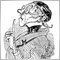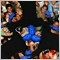# Double Comparison50

Hello

How we can compare double value ,when double value is nearly equal to zero?Moderator
8873

I am using things like this:

```#define isZero(x) ((fabs(x) < 0.000000001)
```2023390

just like that840

Standard numerical comparisons ( >, <, == (logical) <=, >=, and so on) should work.  If you are not getting enough precision with double, then I think you are stuck, because it is even higher precision than float, according to this page.20233

The problem has nothing to do with precision (float vs double.)

The comparators work fine. The problem is with the equals. Is the equality important?

If you code Bid > trigger, is it OK for that to be true when Bid and Trigger are the same price? If you code Bid >= trigger, is it OK for it to be true when Bid is slightly below Trigger because of round off?

```//        1        2        3        4        5        6        7        8        9       10
double t=1/10.0 + 1/10.0 + 1/10.0 + 1/10.0 + 1/10.0 + 1/10.0 + 1/10.0 + 1/10.0 + 1/10.0 + 1/10.0;
Print(t,"=1.0?",t==0); // 0.9999999999999999=1.0?false```
If neither can be true, the equality is important, and you must understand The == operand. - MQL4 forum You can NOT use NormalizeDouble because that will not work on metals, (point != ticksize,) and NormalizeDouble uses Digits.
```if( Bid - trigger >  0          ) // Bid >  trigger or possibly Bid == trigger
if( Bid > trigger               ) // equivalent.

if( Bid - trigger >  ticksize/2 ) // Bid >  trigger
if( Bid - trigger > -ticksize/2 ) // Bid >= trigger
```326

Always make your programmer's life easy. So pick up Carl's valuable tip and write defines in your classes where you need your double comparisons:

```// add more floating point zeros if you need a higher precision
#define isZero(x) (fabs(x) < 0.000000001)
#define isEqual(x,y) (fabs(x-y) < 0.000000001)

```

When you need to compare two doubles then use it in a condition like this:

```if (isEqual(myFirstDouble, mySecondDouble))
{
// first and second doubles are considered equal
// do something
}```

If you want to see if a double is zero (or very, very close to zero) use a condition like this:

```if (isZero(myDouble))
{
// myDouble is considered zero
// do something
}```